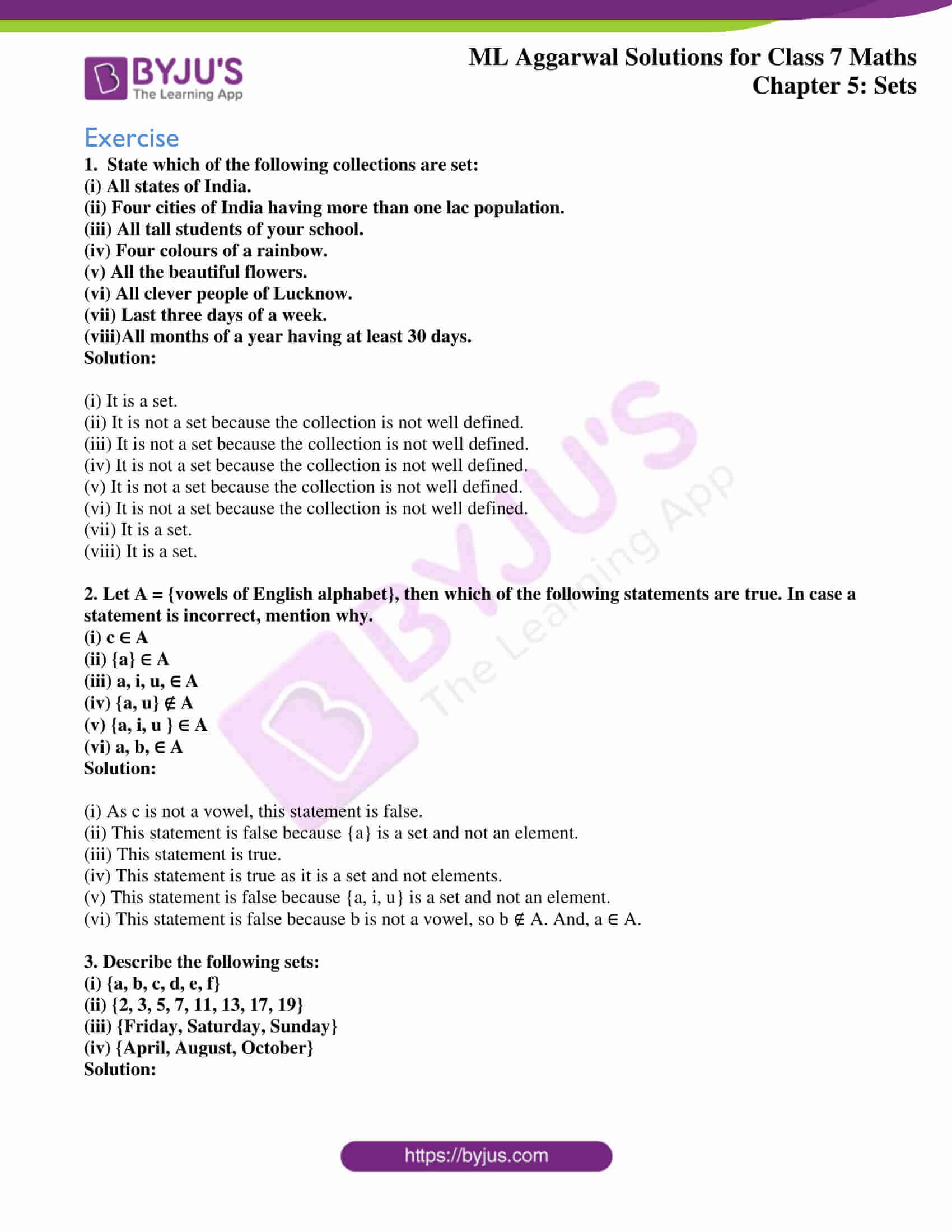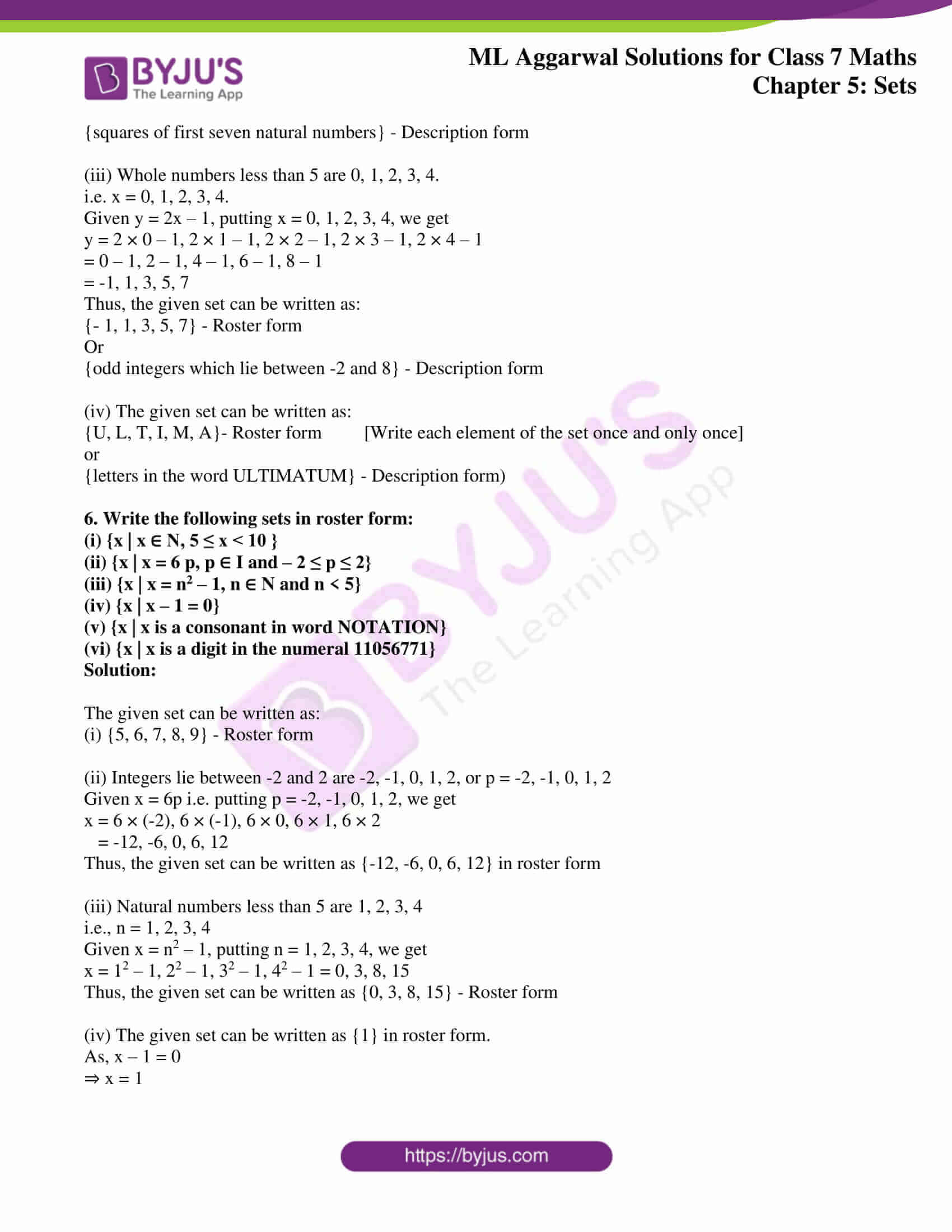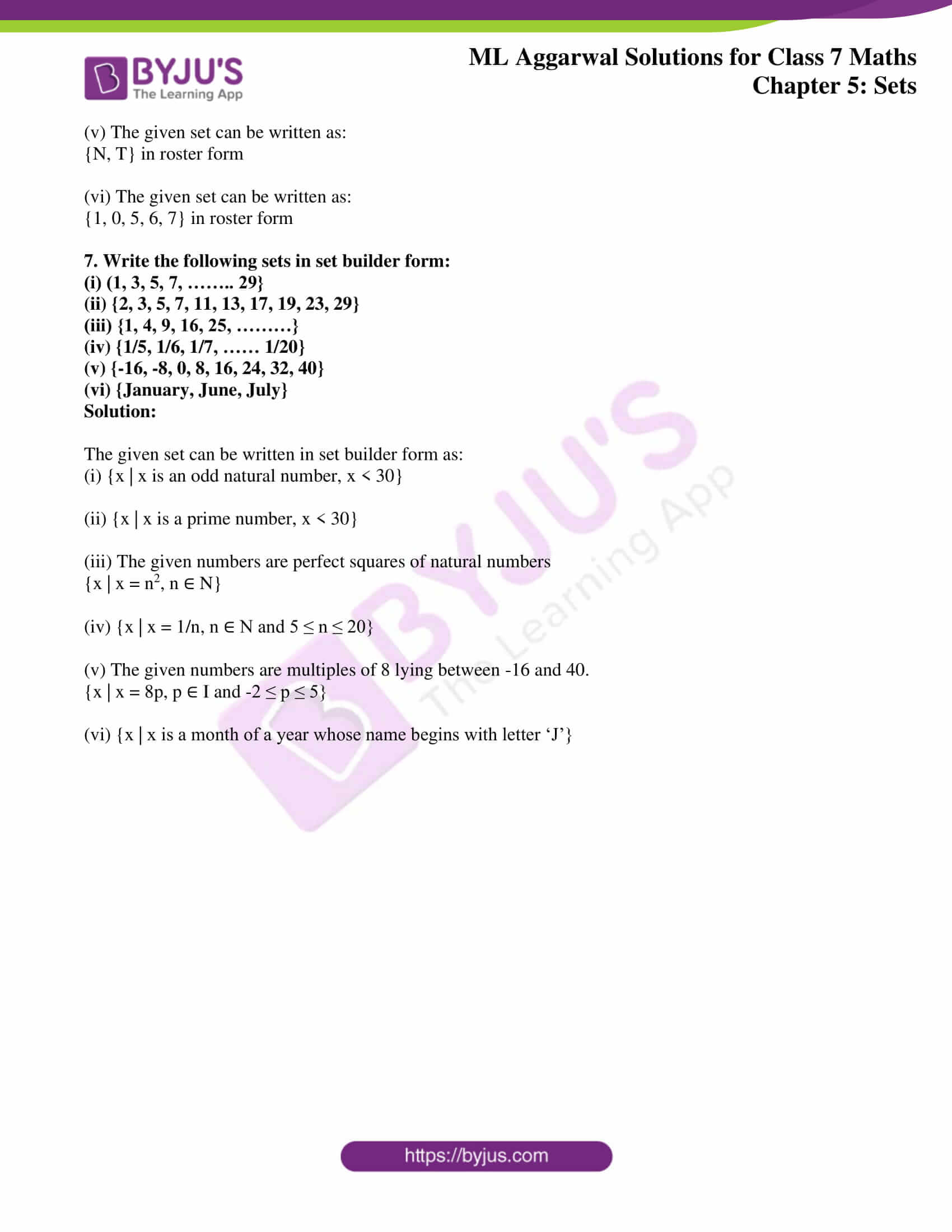# ML Aggarwal Solutions for Class 7 Maths Chapter 5 Sets

ML Aggarwal Solutions for Class 7 Maths Chapter 5 Sets are given here to aid students with their exam preparations. This chapter mainly deals with problems based on the definition of a set and its forms of representation. The solutions are created in an interesting manner in order to make the process of learning more engaging. The ML Aggarwal Solutions is the right resource for students to prepare for their exams effortlessly. Using this resource for practising problems on regular intervals can increase the academic performance of students. Students can now easily download the solutions and can start practising offline at any time and anywhere.

Chapter 5 Sets provides solutions to questions related to the topics covered in this chapter. Students can access and easily download the PDF of ML Aggarwal Class 7 Solutions for free, from the links given below.

## Download the PDF of ML Aggarwal Solutions for Class 7 Maths Chapter 5 Sets### Access answers to ML Aggarwal Solutions for Class 7 Maths Chapter 5 Sets

Exercise

1. State which of the following collections are set:
(i) All states of India.
(ii) Four cities of India having more than one lac population.
(iii) All tall students of your school.
(iv) Four colours of a rainbow.
(v) All the beautiful flowers.
(vi) All clever people of Lucknow.
(vii) Last three days of a week.
(viii)All months of a year having at least 30 days.
Solution:

(i) It is a set.
(ii) It is not a set because the collection is not well defined.
(iii) It is not a set because the collection is not well defined.
(iv) It is not a set because the collection is not well defined.
(v) It is not a set because the collection is not well defined.
(vi) It is not a set because the collection is not well defined.
(vii) It is a set.
(viii) It is a set.

2. Let A = {vowels of English alphabet}, then which of the following statements are true. In case a statement is incorrect, mention why.
(i) c ∈ A
(ii) {a} ∈ A
(iii) a, i, u, ∈ A
(iv) {a, u} ∉ A
(v) {a, i, u } ∈ A
(vi) a, b, ∈ A
Solution:

(i) As c is not a vowel, this statement is false.
(ii) This statement is false because {a} is a set and not an element.
(iii) This statement is true.
(iv) This statement is true as it is a set and not elements.
(v) This statement is false because {a, i, u} is a set and not an element.
(vi) This statement is false because b is not a vowel, so b ∉ A. And, a ∈ A.

3. Describe the following sets:
(i) {a, b, c, d, e, f}
(ii) {2, 3, 5, 7, 11, 13, 17, 19}
(iii) {Friday, Saturday, Sunday}
(iv) {April, August, October}
Solution:

The given set can be written as:
(i) The set of first six letters of alphabet or {first six letters of alphabet}.
(ii) The set of prime numbers less than 20 or {prime numbers less than 20}.
(iii) The set of last three days of a week or {last three days of a week}.
(iv) The set of months of a year whose name begin with a vowel or {months of a year whose name begin with a vowel}.

4. Write the following sets in tabular form and also in set builder form:
(i) The set of even whole numbers which lie between 10 and 50.
(ii) {months of a year having more than 30 days}
(iii) The set of single-digit whole numbers which are a perfect square.
(iv) The set of factors of 36.

Solution:

The given set can be written as:
(i) Tabular form: {12, 14, 16, 18, 20, ……. , 48}
Set builder form: {x : x = 2n, n ∈ N and 5 < n < 25}

(ii) Tabular form: {January, March, May, July, August, October, December)
Set builder form: {x | x is a month of a year having 31 days}

(iii) Tabular form: {0, 1, 4, 9}
Set builder form: {x | x is a perfect square of one digit number)

(iv) Tabular form: {1,2, 3, 4, 6, 9, 12, 18, 36}
Set builder form: {x | x is a factor of 36}

5. Write the following sets in roster form and also in description form:
(i) {x | x = 4n, n ∈ W and n < 5}
(ii) {x : x = n2, n ∈ N and n < 8}
(iii) y : y = 2x – 1, x ∈ W and x < 5}
(iv) {x : x is a letter in word ULTIMATUM}
Solution:

(i) Whole numbers less than 5 are 0, 1, 2, 3, 4.
4n i.e., four times the above numbers are 0, 4, 8, 12, 16.
Thus, the given set can be written as:

{0, 4, 8, 12, 16} – Roster form
Or
{whole numbers which are divisible by 4 and less than 20} – Description form

(ii) Natural numbers less than 8 are 1, 2, 3, 4, 5, 6, 7
n2 i.e., squares of these numbers are 1, 4, 9, 16, 25, 36, 49
Thus, the given set can be written as:

{1,4, 9, 16, 25, 36, 49} – Roster form
Or

{squares of first seven natural numbers} – Description form

(iii) Whole numbers less than 5 are 0, 1, 2, 3, 4.
i.e. x = 0, 1, 2, 3, 4.
Given y = 2x – 1, putting x = 0, 1, 2, 3, 4, we get
y = 2 × 0 – 1, 2 × 1 – 1, 2 × 2 – 1, 2 × 3 – 1, 2 × 4 – 1
= 0 – 1, 2 – 1, 4 – 1, 6 – 1, 8 – 1
= -1, 1, 3, 5, 7
Thus, the given set can be written as:

{- 1, 1, 3, 5, 7} – Roster form
Or
{odd integers which lie between -2 and 8} – Description form

(iv) The given set can be written as:

{U, L, T, I, M, A}- Roster form [Write each element of the set once and only once] or
{letters in the word ULTIMATUM} – Description form)

6. Write the following sets in roster form:
(i) {x | x ∈ N, 5 ≤ x < 10 }
(ii) {x | x = 6 p, p ∈ I and – 2 ≤ p ≤ 2}
(iii) {x | x = n2 – 1, n ∈ N and n < 5}
(iv) {x | x – 1 = 0}
(v) {x | x is a consonant in word NOTATION}
(vi) {x | x is a digit in the numeral 11056771}
Solution:

The given set can be written as:
(i) {5, 6, 7, 8, 9} – Roster form

(ii) Integers lie between -2 and 2 are -2, -1, 0, 1, 2, or p = -2, -1, 0, 1, 2
Given x = 6p i.e. putting p = -2, -1, 0, 1, 2, we get
x = 6 × (-2), 6 × (-1), 6 × 0, 6 × 1, 6 × 2
= -12, -6, 0, 6, 12
Thus, the given set can be written as {-12, -6, 0, 6, 12} in roster form

(iii) Natural numbers less than 5 are 1, 2, 3, 4
i.e., n = 1, 2, 3, 4
Given x = n2 – 1, putting n = 1, 2, 3, 4, we get
x = 12 – 1, 22 – 1, 32 – 1, 42 – 1 = 0, 3, 8, 15
Thus, the given set can be written as {0, 3, 8, 15} – Roster form

(iv) The given set can be written as {1} in roster form.
As, x – 1 = 0

⇒ x = 1

(v) The given set can be written as:

{N, T} in roster form

(vi) The given set can be written as:
{1, 0, 5, 6, 7} in roster form

7. Write the following sets in set builder form:
(i) (1, 3, 5, 7, …….. 29}
(ii) {2, 3, 5, 7, 11, 13, 17, 19, 23, 29}
(iii) {1, 4, 9, 16, 25, ………}
(iv) {1/5, 1/6, 1/7, …… 1/20}
(v) {-16, -8, 0, 8, 16, 24, 32, 40}
(vi) {January, June, July}
Solution:

The given set can be written in set builder form as:
(i) {x | x is an odd natural number, x < 30}

(ii) {x | x is a prime number, x < 30}

(iii) The given numbers are perfect squares of natural numbers
{x | x = n2, n ∈ N}

(iv) {x | x = 1/n, n ∈ N and 5 ≤ n ≤ 20}

(v) The given numbers are multiples of 8 lying between -16 and 40.

{x | x = 8p, p ∈ I and -2 ≤ p ≤ 5}

(vi) {x | x is a month of a year whose name begins with letter ‘J’}